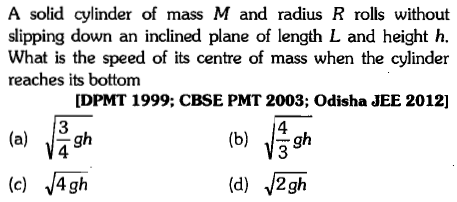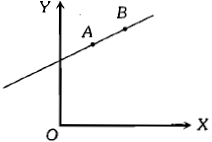A disk and a sphere of the same radius but different masses roll off on two inclined planes Of the same altitude and length Which one of the two objects gets to the bottom of the plane first

1.  Disk

2.   Sphere

3.  Both reach at the same time

4.  Depends on their masses

Concept Questions :-

Rolling motion
High Yielding Test Series + Question Bank - NEET 2020

Difficulty Level:

A ball hits the floor and rebounds after an inelastic collision. In this case

1.  the momentum of the ball just after the collision is the same as that just before the collision

2.  the mechanical energy of the ball remains the same in the collision

3.  the total momentum of the ball and the earth is conserved

4.  the total energy of the ball and the earth is conserved

Concept Questions :-

Linear momentum
High Yielding Test Series + Question Bank - NEET 2020

Difficulty Level:

The rotational KE of a body is E and its moment of inertia is I. The angular momentum is

1.  EI

2.  $2\sqrt{\left(\mathrm{EI}\right)}$

3.

4.  $\frac{\mathrm{E}}{\mathrm{I}}$

Concept Questions :-

Angular momentum
High Yielding Test Series + Question Bank - NEET 2020

Difficulty Level:A solid cylinder of mass M and radius R rolls without slipping down an inclined plane of length L and height h. What is the speed of its centre of mass when the cylinder reaches its bottom

1.  $\sqrt{\frac{3}{4}\mathrm{gh}}$

2.  $\sqrt{\frac{4}{3}\mathrm{gh}}$

3.

4.

Concept Questions :-

Rolling motion
High Yielding Test Series + Question Bank - NEET 2020

Difficulty Level:

A particle of mass m moves in the XY plane with a velocity V along the straight line AB. If the angular momentum of the particle about the origin O is ${\mathrm{L}}_{\mathrm{A}}$ when it is at A and ${\mathrm{L}}_{\mathrm{B}}$ when it is at B, then;1.

2.

3.  The relationship between  depends upon the slope of the line AB

4.

Concept Questions :-

Angular momentum
High Yielding Test Series + Question Bank - NEET 2020

Difficulty Level:

If rotational kinetic energy is 50 % of translational kinetic energy, then the body is

1. Ring

2. Cylinder

3. Hollow sphere

4. Solid sphere

Concept Questions :-

Moment of inertia
High Yielding Test Series + Question Bank - NEET 2020

Difficulty Level:

Consider a system of two identical particles. One of the particles is at rest and the other has an acceleration a. The centre of mass has an acceleration

1. zero

2. 1/2 a

3. a

4. 2 a

Concept Questions :-

Center of mass
High Yielding Test Series + Question Bank - NEET 2020

Difficulty Level:

A solid sphere rolls without slipping down a $30°$ inclined plane. If g = 10 $\mathrm{m}/{\mathrm{s}}^{2}$, the acceleration of the rolling sphere is

1.  5 ${\mathrm{ms}}^{-2}$

2.  $\frac{7}{25}{\mathrm{ms}}^{-2}$

3.  $\frac{25}{7}{\mathrm{ms}}^{-2}$

4.  $\frac{15}{7}{\mathrm{ms}}^{-2}$

Concept Questions :-

Rolling motion
High Yielding Test Series + Question Bank - NEET 2020

Difficulty Level:

If the equation for the displacement of a particle moving on a circular path is given by $\theta =2{t}^{3}+0.5$, where $\theta$ is in radian and t is in second, then the angular velocity of the particle after 2s is

Concept Questions :-

Rotation motion : Introduction
High Yielding Test Series + Question Bank - NEET 2020

Difficulty Level:

A pan containing a layer of uniform thickness of ice is placed on a circular turntable with its centre coinciding with the centre of the turn table. The turntable is now rotated at a constant angular velocity about a vertical axis passing through its centre and then driving is withdrawn. There is no friction between the table. As the ice melts

1.  the angular velocity of the system decreases

2.  the angular velocity of the system increases

3.  the angular velocity of the system remains unchanged

4.  the moment of inertia of the system decreases

Concept Questions :-

Angular momentum